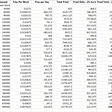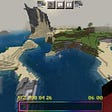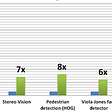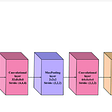# Image Classification Neural Network Tutorial: Getting Started with DL4J

## We’ll look at MINST Digits images dataset to build image classification neural network using DL4J

This is the second article of exploring DL4J library to learn deep learning concepts. In this we’ll work with our first image classification problem.

## Prerequisite:

It is of high importance that you finish this tutorial first:

## Dataset:

We’ll be using a famous dataset called MNIST (Basically the hello world of image classification). General MNIST dataset consist of 70,000 images of 28×28 pixels, representing handwritten 0–9 digits. 60,000 are part of the training set, which is the set used to train the network, while the remaining 10,000 are part of the test set. Download the .zip file:

If you extract it (for now jst extract it in downloads folder as we’re exploring) you can see the dataset is split into two folders: training and testing, each one containing 10 subfolders, labeled 0 to 9, each one in turn containing thousands (almost 6,000) of image samples of handwritten digits correspondent to the label identified by the subfolder name.

Do look at the image information, it has 28x28 dimensions and color space is gray.

## Let’s start coding

As you have already created a project so let’s add a new java class in it named `MinstClassifier`

Before we write any code let’s first add the data in the project. When you extract the `.zip` the file structure is minst_png → training → {Folders from 0 to 9} → {images in folders}. Drag and drop the `minst_png` folder into the resources folder in your project. It’ll copy everything the subfolders and all images as shown below:

`private static final String RESOURCES_FOLDER_PATH = "ADD_PATH_TO_RESOURCE_HERE";private static final int HEIGHT = 28;private static final int WIDTH = 28;private static final int N_SAMPLES_TRAINING = 60000;private static final int N_SAMPLES_TESTING = 10000;private static final int N_OUTCOMES = 10;`

Let’s address the `RESOURCES_FOLDER_PATH` first. In order to add path to resource folder here right click on `mnist_png` folder → copy → Absolute path and paste it in place of `ADD_PATH_TO_RESOURCE_HERE` in the code above.

Image height and width in all folders is 28x28 and there are total 60,000 images combines in all 10 folders and 10,000 in test folder and the outcomes are going to be from 0 to 9 i.e. 10.

Before we create the main function let’s first set our data set iterator method. Next. Create the following function:

`private static DataSetIterator getDataSetIterator(String folderPath, int nSamples) throws IOException {}`

Inside this method we’ll start first by listing the folders 0 to 9. Add this code inside `getDataSetIterator` function.

`File folder = new File(folderPath);File[] digitFolders = folder.listFiles();`

The above code is pretty straight forward, we’ll give it path of folders i.e. training or testing then we’ll get all the sub folders in `digitFolders` which will be an array as there will be 10 subfolder.

Next, we’ll create two objects that will translate help us translate each image into a sequence of 0 to 1 values.

`NativeImageLoader nil = new NativeImageLoader(HEIGHT, WIDTH);ImagePreProcessingScaler scalar = new ImagePreProcessingScaler(0,1);`

NativeImageLoader is responsible to read the image pixels as 0 to 255 integer values. If you know the basics of image processing an image consist of pixels and each pixel has a value from 0 to 255 where 0 indicate black and 255 indicate white. Here is a good link https://www.rapidtables.com/web/color/RGB_Color.html to see the 0–255 values of an RGB image. (Note this dataset we’re working with gracalse images so there will be only one color layer of 0–255 whereas an RGB image has 3 layers i.e. 0–255 values for each layer).

ImagePreProcessingScaler is responsible to scale each of the 0–255 values in a 0–1 (floating point) range for example aa pixel with value of 255 will be 1.

Now we’ll create two Arrays that’ll hold input and output.

`INDArray input = Nd4j.create(new int[]{nSamples, HEIGHT*WIDTH});INDArray output = Nd4j.create(new int[]{nSamples, N_OUTCOMES});`

`Nd4j` is an interface part of DL4J that deals with linear functions of the data. It deals in things like lists, matrices etc. `INDArray` is how we create arrays in DL4J environment. In the above code we are creating two arrays one with the `nSamples` we’ll provide and for training it’s height*width and for testing 10.

Next, we’ll scan each folder for subfolders and then transform labels, extract labels and populate matrices.

`int n = 0;for (File digitFolder: digitFolders) {    int labelDigit = Integer.parseInt(digitFolder.getName());    File[] imageFiles = digitFolder.listFiles();    for (File imgFile : imageFiles) {        INDArray img = nativeImageLoader.asRowVector(imgFile);        scaler.transform(img);        input.putRow(n, img);        output.put(n, labelDigit, 1.0);        n++;    }}`

In the code above we have nested for-loop in place. The first for-loop is picking up each folder one at a time from 0 to 9. The folders are named as per their label i.e. inn folder named 4 all images represent 4 so we’re taking the folder name and storing in after converting to `int`. Then we have a `File[]` array in which we are storing all the files in that folder. After that we run a new for-loop on the picked up folder and inside of this we are converting the image into row vector as need the image representation in mathematical form. Then using the scalar that we set earlier i.e. translating the image into 0…1 range to transform the vectorized image. Now we have an image array which we’ll copy into an input matrix and similarly it is passed to output matrix with label that the image should represent ad lastly, we have a row counter `n` which we increment.

Next we’ll compose the input and output matrices into a dataset. The goal is to build and return a DataSetIterator that our neural network can use. So, add the following code next:

`//Joining input and output matrices into a datasetDataSet dataSet = new DataSet(input, output);//Convert the dataset into a listList<DataSet> listDataSet = dataSet.asList();//Shuffle content of list randomlyCollections.shuffle(listDataSet, new Random(System.currentTimeMillis()));int batchSize = 10;//Build and return a dataset iteratorDataSetIterator dsi = new ListDataSetIterator<DataSet>(listDataSet, batchSize);return dsi;`

Now this method is done and we are ready to add `main()` method and use the `getDataSetIterator` method in our main method. This method is used to make dataset iterator which is used by neural networks. So, let’s make our training dataset iterator. So add the `main()` method:

`public static void main(String[] args) throws IOException {    BasicConfigurator.configure();    }`

Here `BasicConfigurator` is written to automatically configure some DL4J exceptions. Inside the main method after `BasicConfigurator` add:

`long t0 = System.currentTimeMillis();DataSetIterator dataSetIterator = getDataSetIterator(RESOURCES_FOLDER_PATH + "training", N_SAMPLES_TRAINING);buildModel(dataSetIterator);`

Now we can build network. So, it’s time to add `buildModel` method:

`private static void buildModel(DataSetIterator dsi) {    int rngSeed = 123;    int nEpochs = 2;    System.out.printf("Build Model...");    MultiLayerConfiguration conf = new NeuralNetConfiguration.Builder()            .seed(rngSeed)            .updater(new Nesterovs(0.006, 0.9))            .l2(1e-4).list()            .layer(new DenseLayer.Builder()                    .nIn(HEIGHT*WIDTH).nOut(1000).activation(Activation.RELU)                    .weightInit(WeightInit.XAVIER).build())            .layer(new OutputLayer.Builder(LossFunctions.LossFunction.NEGATIVELOGLIKELIHOOD)                    .nIn(1000).nOut(N_OUTCOMES).activation(Activation.SOFTMAX)                    .weightInit(WeightInit.XAVIER).build())            .build();}`

In the code above we have a small and simple neural network that have only one hidden layer with 1000 nodes. You can read more about why I’ve used the RELU and SOFTMAX as activation method or XAVIER weights or NEGATIVELOGLIKELIHOOD as loss function by searching these concepts as you’ll need to do a deep learning course to understand the maths and why we use or where we use them. Here is a good article: https://towardsdatascience.com/complete-guide-of-activation-functions-34076e95d044

Now we can use the network to train the set of images in training folder. So, add the following in `buildModel` function after the above code ends.

`MultiLayerNetwork model = new MultiLayerNetwork(conf);model.init();//Print score every 500 interactionmodel.setListeners(new ScoreIterationListener(500));System.out.print("Train Model...");model.fit(dsi);`

In the code above we have created a variable of `MultiLayerNetwork` and provided it with the configurations of our network. This will build our model and the next step is to see how well our model is performing so we need to perform evaluation. Pur the code inside build model function after the above code lines:

`//EvaluationDataSetIterator testDsi = getDataSetIterator(RESOURCES_FOLDER_PATH+"/testing", N_SAMPLES_TESTING);System.out.print("Evaluating Model...");Evaluation eval = model.evaluate(testDsi);System.out.print(eval.stats());long t1 = System.currentTimeMillis();double t = (double)(t1-t0)/1000.0;System.out.print("\n\nTotal time: "+t+" seconds");`

We start by initializing the path to our testing folder and then provided it to `Evaluation` variable using the `MultiLayerNetwork` model that we have just trained. The last three lines are just telling us how much time it took for this model to get trained and evaluated as some modifications to the configurations might not improve accuracy of the model but can reduce time so it’s good to have the time in check. So, now if you run the code you’ll get this:

Our model is able to perform with 96% accuracy which is a very good accuracy. That’s it you have successfully build a classification neural network and here is the full code of this class:

# Conclusion

So, you have just finished building classification model using DL4J. MINST Digit is the hello world of classification example in deep learning projects and I’ll be writing more classification projects but this will be a prerequisite;

Now using the knowledge you have just acquired you can take a simple dataset with only two classes . If you have see silicon valley you can take the hotdog and not-hotdog dataset and build a neural network around it using the same knowledge you have acquired. Here is a dataset I foud on Kaggle that you can use: https://www.kaggle.com/dansbecker/hot-dog-not-hot-dog (Be mindful of the fact that in this article we have worked on grayscale color images and this dataset consist of RGB color images).

Do press the 👏 so that others can find it as well and do highlight if you find any typos.

## More from DataCTW

DataCTW is a website of online tutorials hosted at different places and are completely free. Our aim is to provide tutorials from scratch so that anyone who is looking to learn something new can benefit from it.

## 🍟\$FRIES Token Emission Schedule## Static vs. Dynamic Libraries## The Quickest Guide to Quitting Games in Unity## Developer Incentives in Sia## FIRST Teach Challenge — An Introduction to GitHub## Bedrock Biome Expansion V1.0## Game Changer: Procyon (Part 1)## Hi everyone,## OpenCV using Python## Multi-Class Image Classification with TensorFlow## Facial Expressions Recognition using Keras## History of Object Detection PART1 -HAAR Features